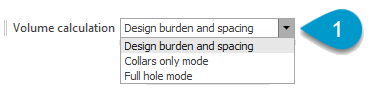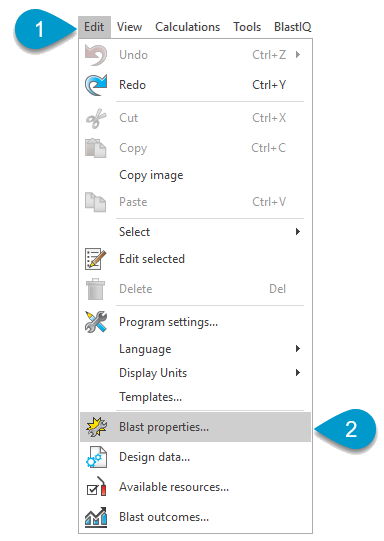# Volume - Calculation Mode in SHOTPlus™SHOTPlus™ Variant:
Surface
Version:
6.14.13 (Beta) and 6.14.2 (Production).
KBA Summary:
This Knowledge Base Articles (KBA) describes the different Volume Calculation modes in SHOTPlus™ and their effect on different parts of the application.

### Background

SHOTPlus™ calculates volume on a hole-by-hole basis. These calculations are done concurrently with user inputs, to ensure that the correct volume is reported. For SHOTPlus™ files with a large number of holes, these calculations can be significantly computationally intensive and can impact the responsiveness of the application.

SHOTPlus™ enables three different modes for these concurrent volume calculations, so that when users are conducting tasks that don't require high precision volume calculations, the mode can be changed to improve performance of the application. These modes are:

1. Design Hole Dimensions - least computationally intensive.
2. Collar Voronoi - moderately computationally intensive.
3. Full Hole Voronoi - most computationally intensive.

### Calculation Mode Formula and Uses

Design Hole Dimensions
Calculation Uses

This mode uses the Hole Type 'Default' Burden and Spacing to define the area for each hole. This area is then used to calculate the volume for the hole.

Hole Area Formula

Hole Collar Area = 'Hole Type Default Burden' x 'Hole Type Default Spacing'

Where:

• 'Hole Type Default Burden' =  'Default' Burden defined for the hole type in 'Edit' > 'Design Data'.
• 'Hole Type Default Spacing' =  'Default' Spacing defined for the hole type in 'Edit' > 'Design Data'.

Hole Volume Formula

Hole Volume = Hole Collar Area x h

Where:

• h = Collar Z - Grade Z.
• If Grade Z = 'null' then h = Collar Z - Toe Z.
Collar Voronoi
Calculation Uses

This mode uses a modified Voronoi Tessellation to calculate the Hole Collar Area for each hole. This area is then used to calculate the volume for each hole.

Hole Volume Formula

Hole Volume = Hole Collar Area x h

Where:

• Hole Collar Area = A hole's collar area calculated using voronoi tessellation.
• h = Collar Z - Grade Z.
• if Grade Z = 'null' then h = Collar Z - Toe Z.
Full Hole Voronoi
Calculation Uses

This mode uses a modified voronoi tessellation to calculate the Hole Collar Area and the Hole Toe Area for each hole. The average of these two areas is then used to calculate the volume for each hole. This is also the mode used by BlastIQ™ Insights to calculate hole volumes.

Hole Volume Formula

Hole Volume = ((Hole Collar Area + Hole Toe Area)/2) X h

Where:

• Hole Collar Area = Collar area calculated using Voronoi Tessellation.
• Hole Toe Area= Toe area calculated using Voronoi Tessellation.
• h = Collar Z - Grade Z.
• if Grade Z = 'null', then h = Collar Z - Toe Z.

### Impact

The volume calculation mode selected, will effect the volumes reported by SHOTPlus™ in the following areas of the application:

1. Analytics View.
2. Volume Overview.
3. Reporting.
4. Blast Summary Block.

The mode selected in SHOTPlus™ DOES NOT affect how volumes are reported in BlastIQ™.

### Selection

Users can select which volume calculation should be used for each file, by:

• Selecting their preferred mode from the down dialog on the toolbar; or• Selecting Edit > Blast Properties from the main menu, then selecting Volume calculations from Edit Blast Properties dialog sidebar, and selected their preferred mode volume calculation modes.